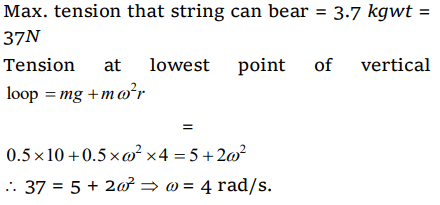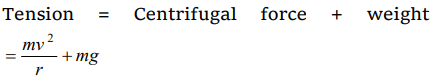## Motion in a Plane Questions and Answers Part-14

1. A weightless thread can bear tension upto 3.7 kg wt. A stone of mass 500 gms is tied to it and revolved in a circular path of radius 4 m in a vertical plane. If $g=10ms^{-2}$  , then the maximum angular velocity of the stone will be
c) $\sqrt{21}$  radians/sec

Explanation:2. The maximum velocity at the lowest point, so that the string just slack at the highest point in a vertical circle of radius l
a) $\sqrt{gl}$
b) $\sqrt{3gl}$
c) $\sqrt{5gl}$
d) $\sqrt{7gl}$

Explanation: $\sqrt{5gl}$

3. If the equation for the displacement of a particle moving on a circular path is given by $\left(\theta\right)=2t^{3}+0.5$   , where $\theta$ is in radians and t in seconds, then the angular velocity of the particle after 2 sec from its start is

Explanation:4. A body of mass m hangs at one end of a string of length l, the other end of which is fixed. It is given a horizontal velocity so that the string would just reach where it makes an angle of $60^{\circ}$ with the vertical. The tension in the string at mean position is
a) 2mg
b) mg
c) 3mg
d) $\sqrt{3}$ mg

Explanation:5. In a vertical circle of radius r, at what point in its path a particle has tension equal to zero if it is just able to complete the vertical circle
a) Highest point
b) Lowest point
c) Any point
d) At a point horizontally from the centre of circle of radius r

Explanation: Highest point

6. The tension in the string revolving in a vertical circle with a mass m at the end which is at the lowest position
a) $\frac{mv^{2}}{r}$
b) $\frac{mv^{2}}{r}-mg$
c) $\frac{mv^{2}}{r}+mg$
d) mg

Explanation:7. A hollow sphere has radius 6.4 m. Minimum velocity required by a motor cyclist at bottom to complete the circle will be
a) 17.7 m/s
b) 10.2 m/s
c) 12.4 m/s
d) 16.0 m/s

Explanation:8. A pendulum bob on a 2 m string is displaced $60^{\circ}$ from the vertical and then released. What is the speed of the bob as it passes through the lowest point in its path
a) $\sqrt{2}$ m/s
b) $\sqrt{9.8}$ m/s
c) 4.43 m/s
d) $1/\sqrt{2}$ m/s

Explanation:9. A fan is making 600 revolutions per minute. If after some time it makes 1200 revolutions per minute, then increase in its angular velocity is
a) $10\pi rad \diagup sec$
b) $20\pi rad \diagup sec$
c) $40\pi rad \diagup sec$
d) $60\pi rad \diagup sec$